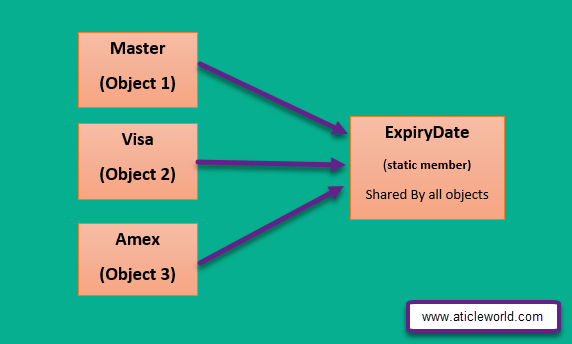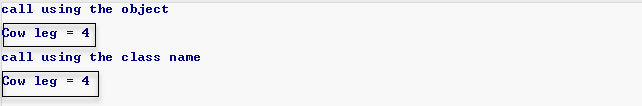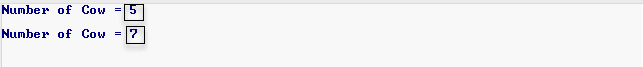# Static Member Function and VariableSometimes in the program, we need to share a memory block with all objects of the class, we can accomplish this requirement using the global variable but we know that global variable is not much safer its value can be modified by anyone in the program.

So to solve this problem, C++ provides a solution, If we qualified a member variable with the static keyword then it will alive throughout the program and its value would be shared by all objects of that class because static member variable not associated with the class objects it’s associated with the class.

Hence sometimes people refer static member variable as the class variable. When you create a static member variable in the class then does not any storage space allocated to this variable, you have to define it explicitly outside of the class.

If you forget to define the static member variable outside of the class then you will get the linker error.

In below class template Date is static member variable it is associated with Journey class, if you want to access the Date member variable then you have to define it explicitly outside of the class.

### Syntax to define the static member variable

We can define the static member in c++ using the class name and resolution operator, see the below syntax.

Using the above syntax, we can define the Date (static member variable) variable outside of the class, see the below code.

#### Real life example of static member variable in C++

Information.h

Implementation of file, Information.cppThe image describes, how static member variable shares with class objects.

In the above code, we make the ExpiryDate as the static member because it accesses by more than one modules in the program.

We already know, when we create the object of CardInformation class then memory is allocated to its members except for the static members because static members are not associated with objects, it is associated with class. So here ExpiryDate is initialized in the code outside of the class, if we do not do that then we get the linker error.

Some people say that why we are not able to define static member within the class?
It’s very interesting question and its answer is so simple actually, C++ follow the one definition law through which a transaction unit only contain a single definition.

In case of static member variable it shared by all objects which created from the class, so a static member variable have more than one definition which is not allowed in c++, that is the reason we have to define static member outside of the class but there is an exception we can initialize an integral static constant variable within the class.

Some valid member variables of C++.

#### Some important points of static member variable

• In the program, static member variable creates once for a class and it can be public or private.
• There is only one copy create to the static member variable and these copy shared by all object.
• We cannot define a static variable within class only define outside of class.
• Static member variable can be accessed through class name using the resolution operator.
• Static member variable can be initialized in the class but when it is qualified by the const keyword and variable must be the integral type.

#### Need of static member variable in C++

We already know that when we qualify a variable with the static keyword then we are able to share this variable with all created objects and the static variable does not depend on the objects.
Sometimes in the program when we need to call a variable without the creating the object, this requirement is fulfilled by the static variable. We can call the static variable with their class name using the resolution operator (::) . In below I am describing the example which describes the importance of the static variable.The code explains, how to access the leg a static member variable using the object and class name.

#### Use of static member function in C++

When we make a static member variable private then it only accesses by the class member function.

See the below example code.The code explains how to access the private static member variable using the member function. Everything is ok here but problem is that to access the member function we need to create the object of Animal class but, we don’t want to create the object of Animal class.
This issues will be solved when we qualify the member function with static keyword, we can also call the static member function with their class name using the resolution operator (::).The code explains, how to access the static private member variable and member function using the class name and resolution operator.

#### Some important points of static member function

• A “this” pointer does not associate with the static member function.

OutPut:  [Error] ‘this’ is unavailable for static member functions

• A static member function does not have this pointer, so there is no meaning of using a CV qualifier (const, volatile, const volatile) with static member function because cv-qualifier modify the behavior of this pointer.

E.g. When to use const with member function then the type of this pointer is const *.Static member function only accesses static member variable and another variable outside of the class.

When we compile the above code we get the compiler error, because we are using here const with the static Sound member function.

• A static member function can be access through the class name using resolution operator.
• We cannot overload a static and non-static member function which have the same name, same number and the type of argument.

When compile the above code we get the compiler error because here we try to overload the sound static member function.

• There is no need to write static when we define static member function outside of the class.
• The static member in c++ should not be virtual.